• ## 表示学习

千次阅读 2018-05-15 17:40:44
表示学习——回顾与展望摘要——机器学习算法的成功通常取决于数据表示，并且我们假设这是因为不同的表示可能会或多或少地隐藏和隐藏数据背后变化的不同解释因素。尽管可以使用特定的领域知识来帮助设计表示，但也...
表示学习——回顾与展望摘要——机器学习算法的成功通常取决于数据表示，并且我们假设这是因为不同的表示可能会或多或少地隐藏和隐藏数据背后变化的不同解释因素。尽管可以使用特定的领域知识来帮助设计表示，但也可以使用通用的先验知识进行学习，而对AI的追求则激励设计更强大的表示学习算法来实现这样的先验知识。 本文回顾了近期在无监督特征学习和深度学习方面的工作，包括概率模型，自动编码器，流形学习和深度网络。这激发了关于学习良好表示，计算表示（即推论）以及表示学习，密度估计和流形学习之间的几何关系的长期未解答的问题。 关键词——深度学习，表示学习，无监督学习，玻尔兹曼机，自编码，神经网络 1. 导论机器学习方法的性能在很大程度上取决于它们所应用的数据表示（或特征）的选择。出于这个原因，部署机器学习算法的实际工作大部分都集中在预处理流水线和数据转换的设计上，导致数据表示可以支持有效的机器学习。这样的特征工程很重要，但是需要大量劳动力，突出了当前学习算法的弱点：他们无法从数据中提取和组织信息。特征工程是一种利用人类独创性和先前知识来弥补这一弱点的方法。为扩大机器学习的适用范围和易用性，非常希望使学习算法更少地依赖于特征工程，从而可以更快地构建新的应用程序，更重要的是，可以朝向人工智能（AI）迈进。人工智能必须从根本上理解我们周围的世界，并且我们认为只有当它能够学会识别和分解隐藏在观察到的低级别感官数据环境中的潜在解释性因素时才能实现。本文关于表示学习，即学习数据的表示，以便在构建分类器或其他预测器时更容易提取有用的信息。在概率模型的情况下，好的表示通常是捕获观察输入的基本解释性因素的后验分布。一个好的表示也是一个有用的监督预测器的输入。在各种学习表征方式中，本文重点介绍深度学习方法：通过多个非线性变换的组合形成的方法，其目标是产生更抽象的，最终更有用的表示。在这里，我们调查这个快速发展的地区，特别强调最近的进展。我们考虑一些推动该领域研究的基本问题。具体来说，是什么让一个表示比另一个更好？举一个例子，我们应该如何计算它的表示，即执行特征提取？另外，学习好的表示有什么合适的目标？ 2. 为什么我们应该关注表示学习？2.1   语音识别和信号处理2.2   目标识别2.3   自然语言处理（NLP）2.4   多任务迁移学习，领域适应 3. 怎样才能有好的表示3.1   AI中表示学习的先验知识3.2   平滑度与维数灾难3.3   分布式表示3.4   深度与抽象3.5   解决变异因子（Disentangling Factors of Variation）3.6   表示学习的好的标准4. 建立深度表示5. 单层次学习模型5.1 主成分分析（PCA）6. 概率模型6.1   有向图模型6.1.1 概率挤压（Explaining Away）6.2   无向图模型6.3   受限玻尔兹曼机（RBM）到真值数据的泛化6.4   RBM参数评估6.4.1  CD评估6.4.2  SML算法6.4.3  伪相似性，比率匹配等7. 直接学习从输入到表示的映射参数7.1   自编码7.2   规范化自编码7.2.1  稀疏自编码7.2.2  DAEs7.2.3  CAEs7.2.4  PSD8. 流形学习的表示学习8.1   基于邻居图学习一个参数映射8.2   学习表示非线性流形8.3   扩充模式化正切空间9. 概率模型和直接编码模型的联系9.1   PSD：概率解释9.2   用规范化自编码捕获维度的局部结构9.3   学习近似推理9.4   抽样挑战9.5   评估与监测性能10.深度模型的全局训练10.1  训练深度构架的挑战10.2  DBMs的联合训练10.2.1 平均场的近似推理10.2.2 训练DBMs11.基于不变性11.1  生成变形的实例11.2  卷积和池化11.2.1 基于块的训练11.2.2 卷积和平铺卷积训练11.2.3 转换成池化11.3  时序耦合和慢特征11.4  解决变异因子的算法12.结论   参考文献Representation Learning:A Review and New PerspectivesYoshuaBengio, Aaron Courville, and Pascal Vincent.IEEE TPAMI VOL. 35, NO. 8,AUGUST 2013
展开全文深度学习 无监督学习 神经网络
• ## 表示学习(Representation Learning)

万次阅读 多人点赞 2019-05-10 14:07:39
2013年，Bengio等人发表了关于表示学习的综述。最近拜读了一下，要读懂这篇论文还有很多文献需要阅读。组会上正好报了这篇，所以在此做一个总结。 二、表示学习发展由来 当我们学习一个复杂概念时，总想有一条...
 一、前言

2013年，Bengio等人发表了关于表示学习的综述。最近拜读了一下，要读懂这篇论文还有很多文献需要阅读。组会上正好报了这篇，所以在此做一个总结。

鉴于大家都想要我的汇报PPT，那我就分享给大家，希望能对大家有所帮助。

链接：https://pan.baidu.com/s/1agzlbWy5RLf1zZ7Ojduvvg
提取码：196p

二、表示学习发展由来

当我们学习一个复杂概念时，总想有一条捷径可以化繁为简。机器学习模型也不例外，如果有经过提炼的对于原始数据的更好表达，往往可以使得后续任务事倍功半。这也是表示学习的基本思路，即找到对于原始数据更好的表达，以方便后续任务（比如分类）。人工智能——>机器学习——>深度学习发展经历了一个波折上升的过程，越来越多的模型被发明出来，但想要好的表示效果，还得看数据，数据质量好，数据特征好才是王道。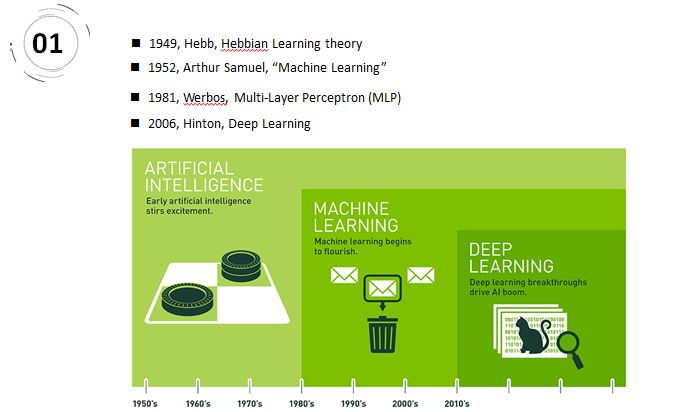赫布于1949年基于神经心理的提出了一种学习方式，该方法被称之为赫布学习理论。2、1952，IBM科学家亚瑟·塞缪尔开发了一个跳棋程序。该程序能够通过观察当前位置，并学习一个隐含的模型，从而为后续动作提供更好的指导。提出机器学习。3、伟博斯在1981年的神经网络反向传播(BP)算法中具体提出多层感知机模型。重新点燃机器学习之火。4、神经网络研究领域领军者Hinton在2006年提出了神经网络Deep Learning算法，使神经网络的能力大大提高，向支持向量机发出挑战。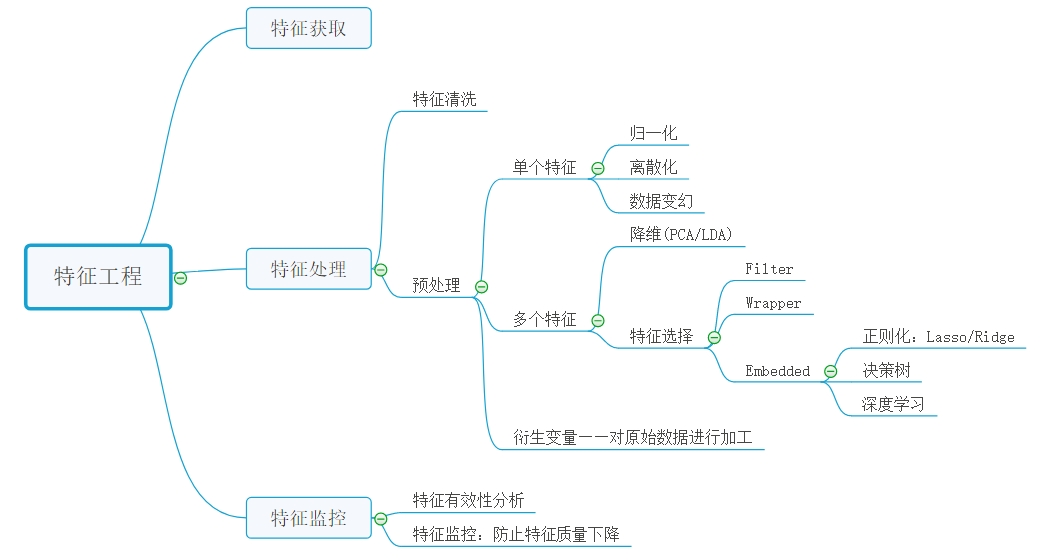“数据决定了机器学习的上限，而算法只是尽可能逼近这个上限”，这里的数据指的就是经过特征工程得到的数据。特征工程就是一个把原始数据转变成特征的过程，这些特征可以很好的描述这些数据，并且利用它们建立的模型在未知数据上的表现性能可以达到最优（或者接近最佳性能）。从数学的角度来看，特征工程就是去设计输入变量X。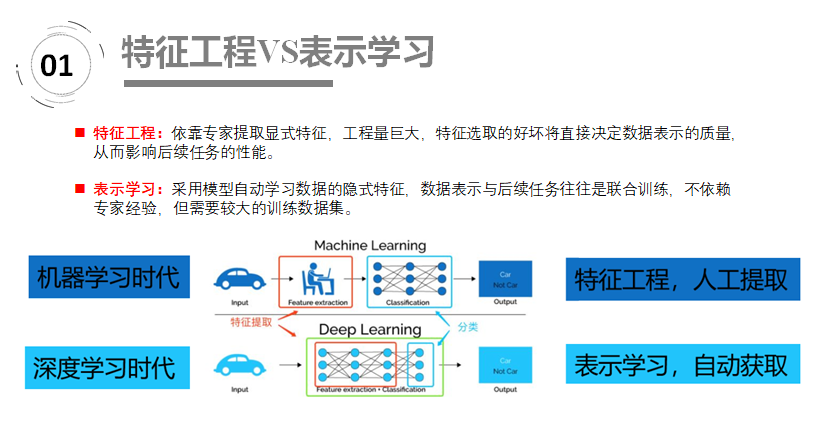在机器学习时代，我们如果需要对汽车进行表示，往往依靠的是领域专家手工提取特征并表示；在深度学习时代，我们直接将汽车输入模型，汽车将自动转换成高效有意义的表示。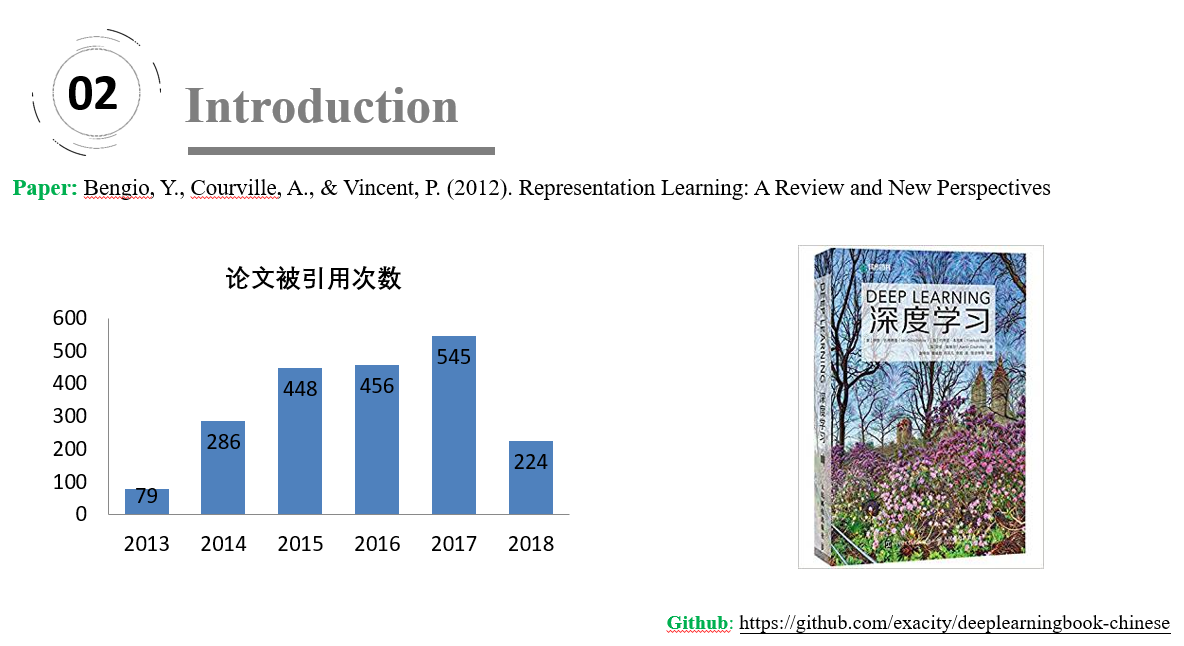2019年3月27日 ——ACM宣布，深度学习的三位创造者Yoshua Bengio， Yann LeCun， 以及Geoffrey Hinton获得了2019年的图灵奖。

三、论文结构

本文回顾非监督特征学习和深度学习领域的一些近期工作，包括概率模型的发展、自动编码机、流行学习和深度网络。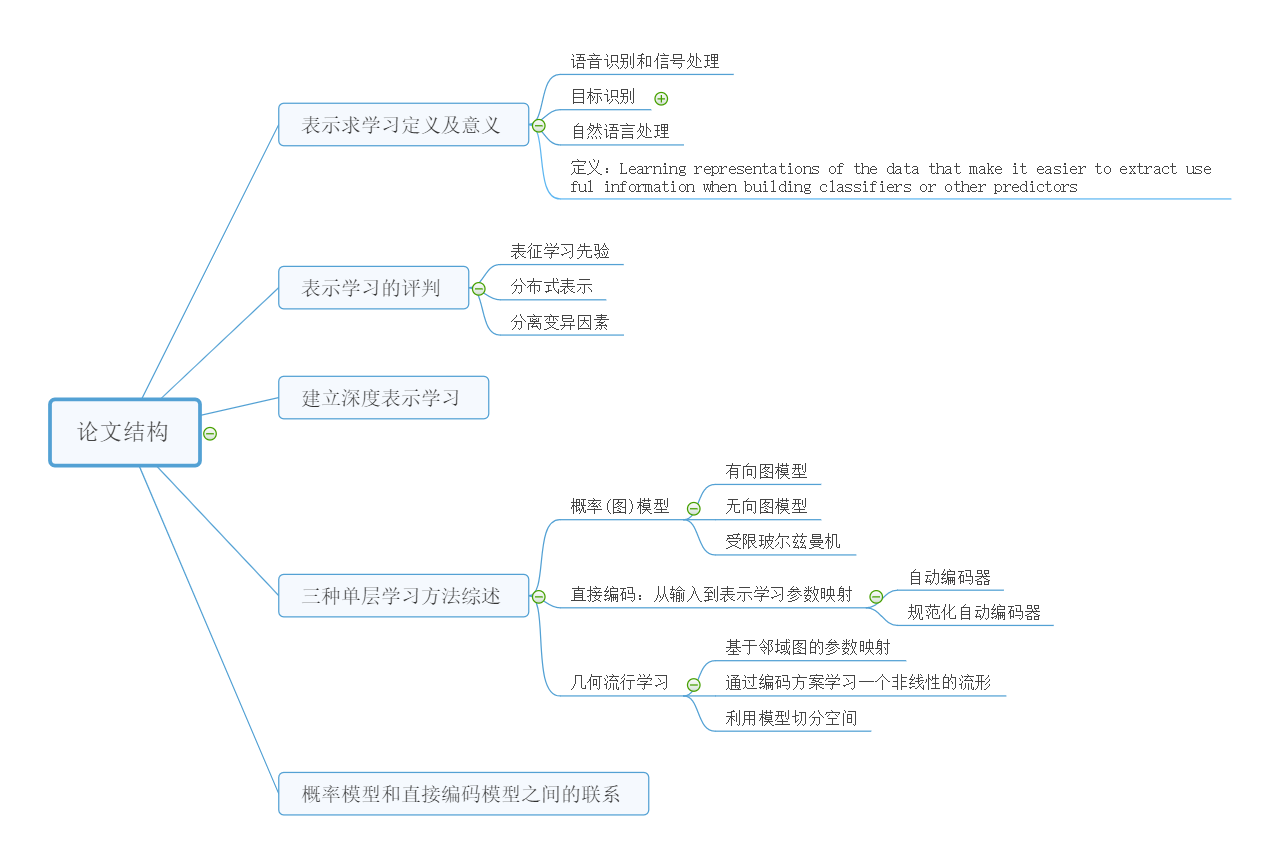定义：Learning representations of the data that make it easier to extract useful information when building classifiers or other predictors。翻译：表示学习以便在构建分类器或其他预测器时更容易提取有用的信息。

分布式表示(distributed representation). 这是深度学习最重要的性质。举一个非常简单的例子，假设我们的词典上有16个词，如果用传统的bag-of-words 的表示方法，我们可以用16维的向量来表示每个词，向量的每一位代表某个词的出现与否。然而，如果我们用分布式表示的思想，我们也可以用四维的向量来代表每一个词，例如(0,0,0,1)， (0,0,1,0),..., (1,1,1,1) 。 这个简单的例子其实用来说明：对同一个输入，我们可以有不同的配置(configuration)。

四、三种单层表示学习方法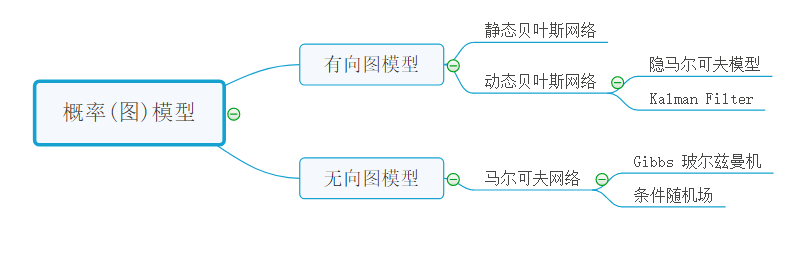概率图模型中的术语图指的是图论，也就是带有边和点的数学对象。确切地说，概率图模型（Probabilistic Graphical Models，PGM）是指：你想描述不同变量之间的关系，但是，你又对这些变量不太确定，只有一定程度的相信或者一些不确定的知识。

贝叶斯概率图模型是有向图，因此可以解决有明确单向依赖的建模问题，而马尔可夫概率图模型是无向图，可以适用于实体之间相互依赖的建模问题。这两种模型以及两者的混合模型应用都非常广泛。条件随机场（CRF）广泛应用于自然语言处理（如词性标注，命名实体识别）。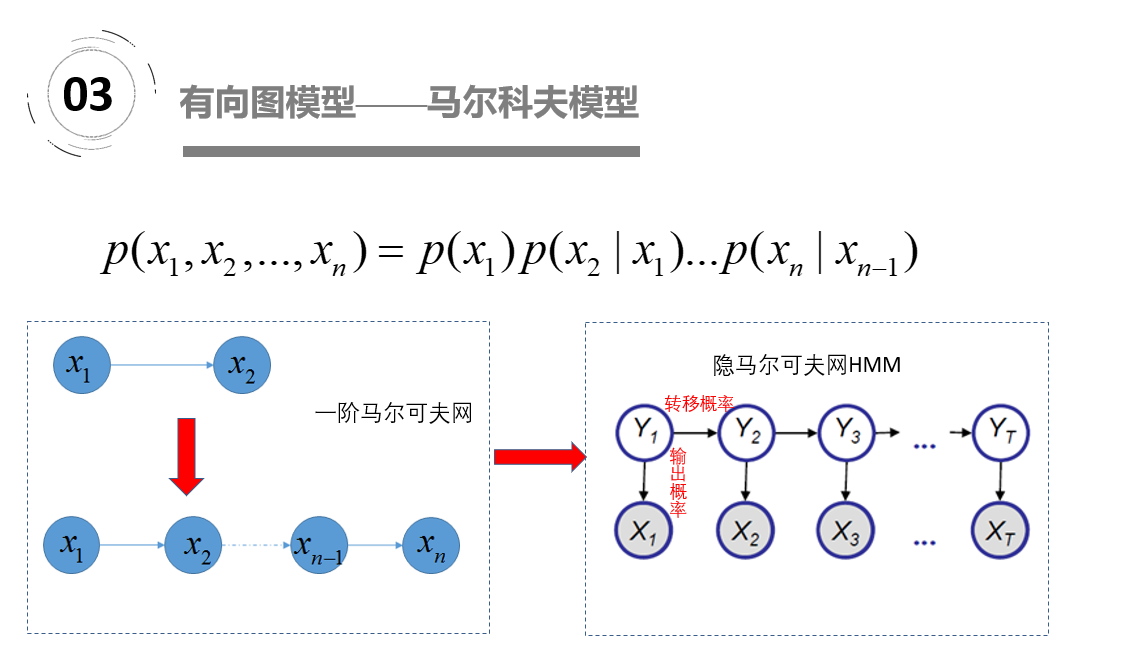在马尔科夫链中，每一个圆圈代表相应时刻的状态，有向边代表了可能的状态转移，权值表示状态转移概率。 HMM，隐马尔可夫模型，是一种有向图模型。由1阶马尔可夫模型演变而来，不同之处在于我们能够观测到的量不是过程本身，而是与其有一定关系的另一些量。那HMM中的隐体现在哪呢？这里“隐”指的是马尔科夫链中任意时刻的状态变量不可见，也就是说状态序列Y1,Y2,...,,...,Yt无法直接观测到。但是HMM中每时刻有一个可见的观测值Xt与之对应.隐马尔可夫模型（HMM）是语音识别的支柱模型.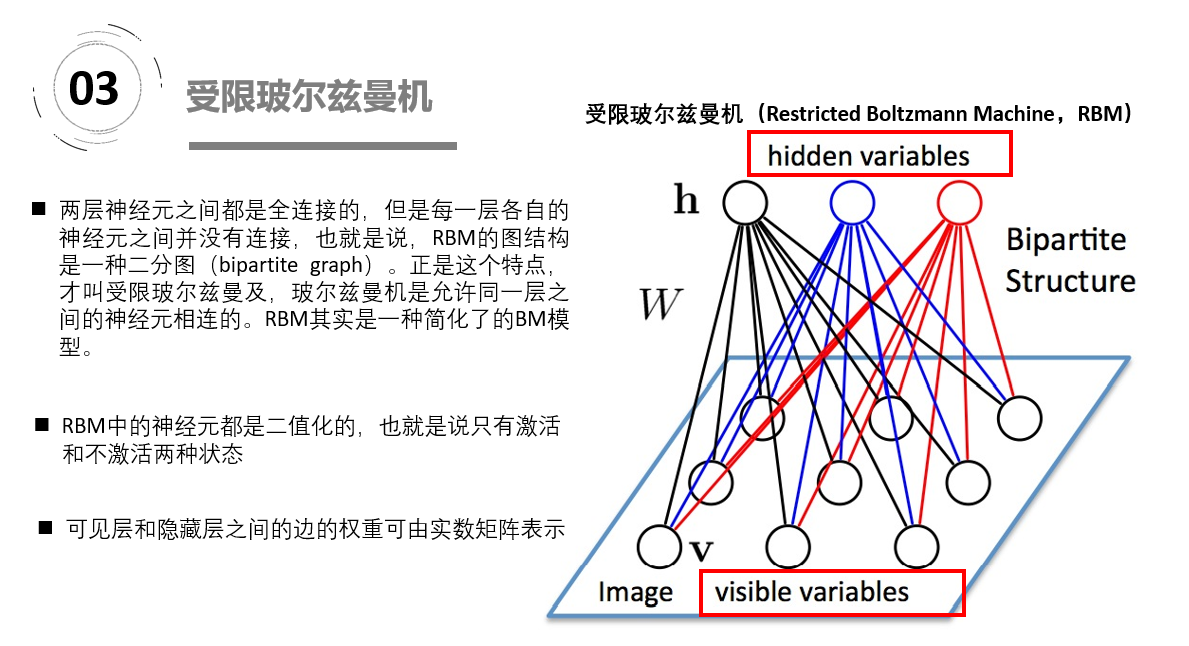玻尔兹曼机是一大类的神经网络模型，但是在实际应用中使用最多的则是受限玻尔兹曼机（RBM）。受限玻尔兹曼机(RBM)能学习并发现数据的复杂规则分布，将多个RBM堆叠就构成了深度置信网络(deep belief network, DBN)，从而可以从更加复杂的高维输入数据中抽取维数更低、区别度较高的特征。https://blog.csdn.net/u013631121/article/details/76652647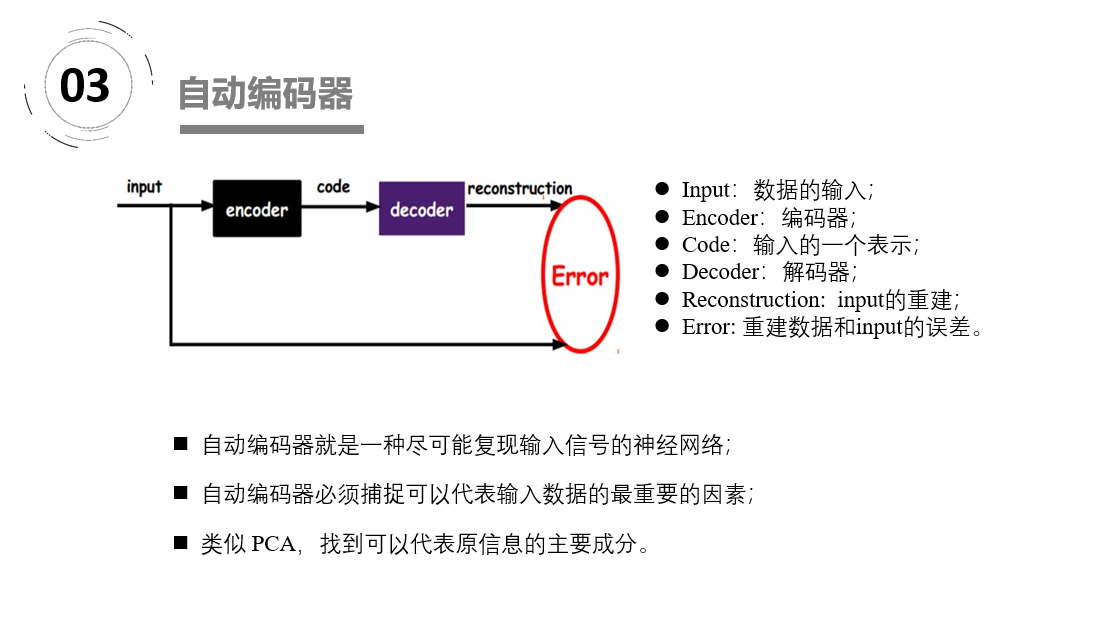自动编码器文章：https://blog.csdn.net/u011584941/article/details/72673260

如上图，我们将input输入一个encoder编码器，就会得到一个code，这个code也就是输入的一个表示，那么我们怎么知道这个code表示的就是input呢？我们加一个decoder解码器，这时候decoder就会输出一个信息，那么如果输出的这个信息和一开始的输入信号input是很像的（理想情况下就是一样的），那很明显，我们就有理由相信这个code是靠谱的。所以，我们就通过调整encoder和decoder的参数，使得重构误差最小，这时候我们就得到了输入input信号的第一个表示了，也就是编码code了。因为是无标签数据，所以误差的来源就是直接重构后与原输入相比得到。

1、稀疏自编码：减小编码后隐藏层神经元个数。2、栈式自编码神经网络是一个由多层稀疏自编码器组成的神经网络，其前一层自编码器的输出作为其后一层自编码器的输入。采用逐层贪婪训练法进行训练，获取栈式自编码神经网络参数。3、去噪自动编码器DA是在自动编码器的基础上，训练数据加入噪声，所以自动编码器必须学习去去除这种噪声而获得真正的没有被噪声污染过的输入。所以他的泛化能力强。4、DAE是通过对输入添加随机噪声，经过编码解码来获得健壮的重构；而CAE对扰动的健壮性是通过惩罚 雅克比矩阵F范数各元素的和得到。CAE抓住内部因素提高特征提取健壮性，DAE通过外部因素提高特征提取健壮性。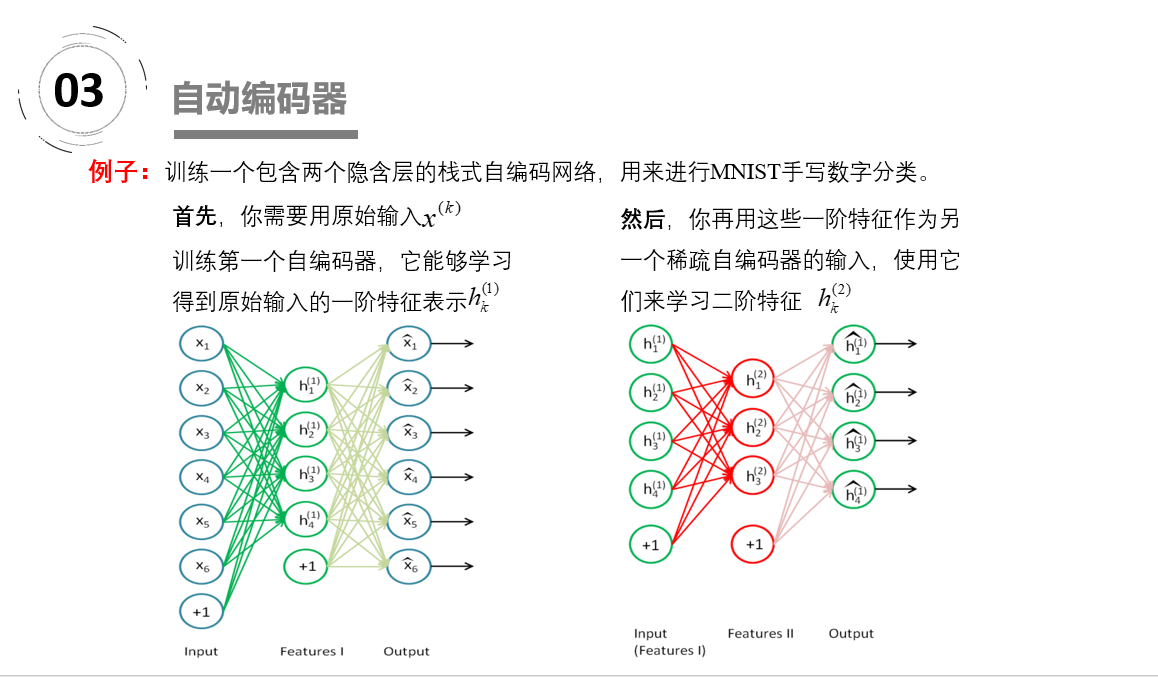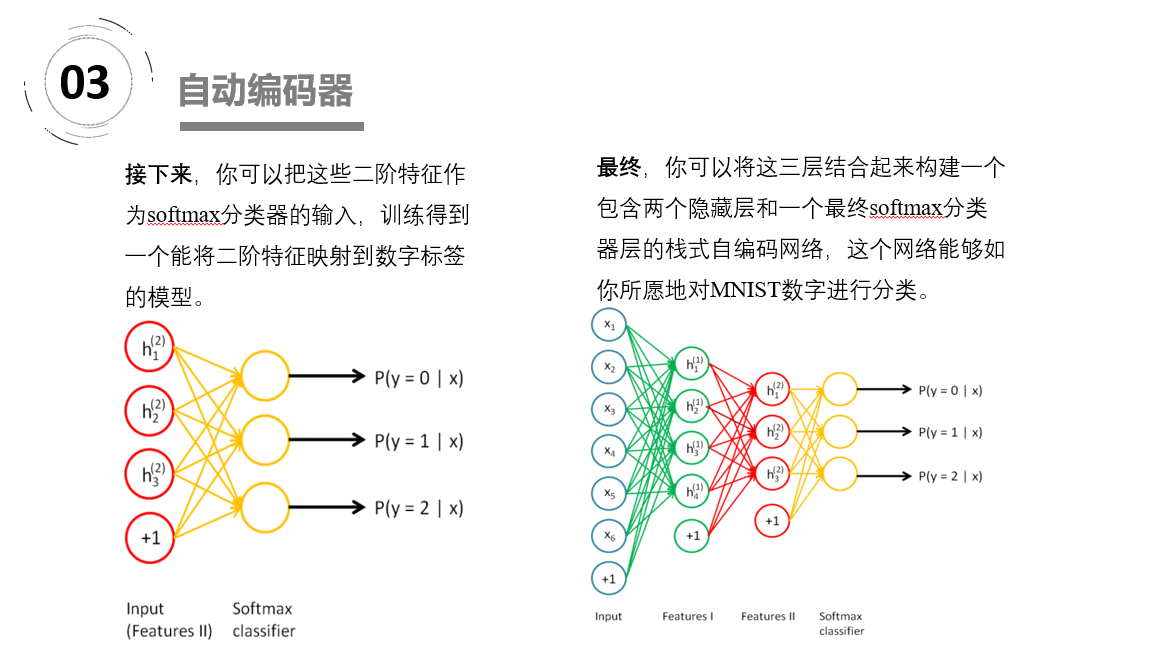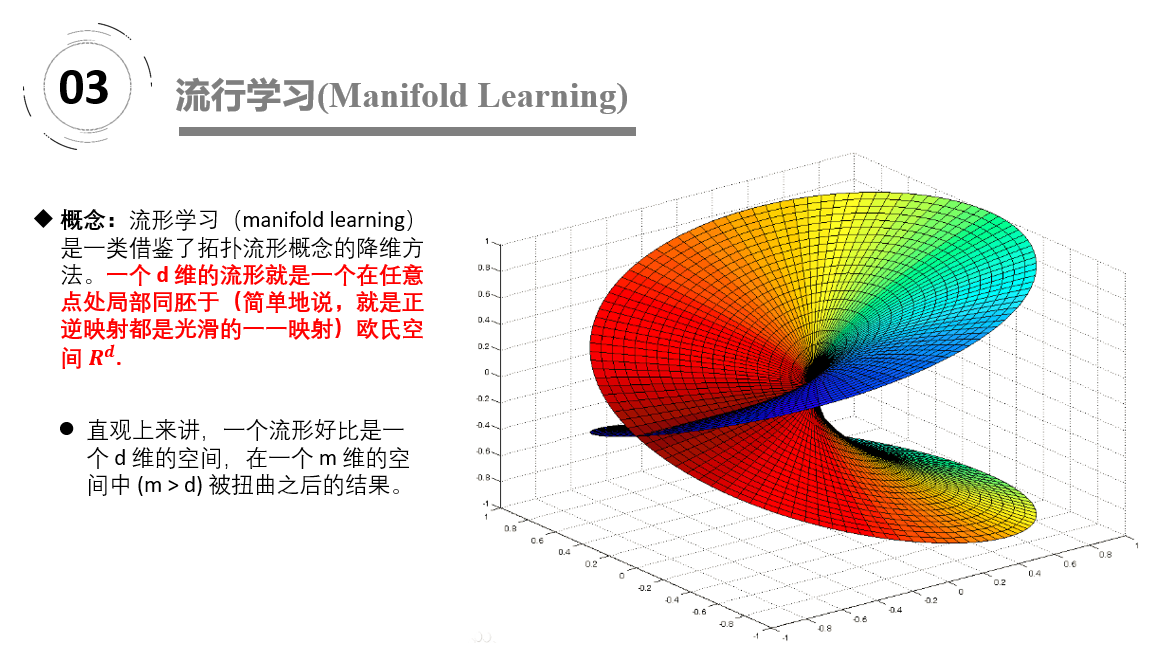“流形”是在局部与欧式空间同胚的空间，换言之，它在局部具有欧式空间的性质，能用欧氏距离来进行距离计算。这给降维方法带来了很大的启发：若低维流形嵌入到高维空间中，则数据样本在高维空间的分布虽然看上去非常复杂，但是在局部上仍具有欧式空间的性质，因此，可以容易地在局部建立姜维映射关系，然后在设法将局部映射关系推广到全局。当维数被将至二维或三维时，能对数据进行可视化展示，因此流行学习也可以被用于可视化。直观上来讲，一个流形好比是一个 d 维的空间，在一个 m 维的空间中 (m > d) 被扭曲之后的结果。广义相对论似乎就是把我们的时空当作一个四维流（空间三维加上时间一维）形来研究的，引力就是这个流形扭曲的结果。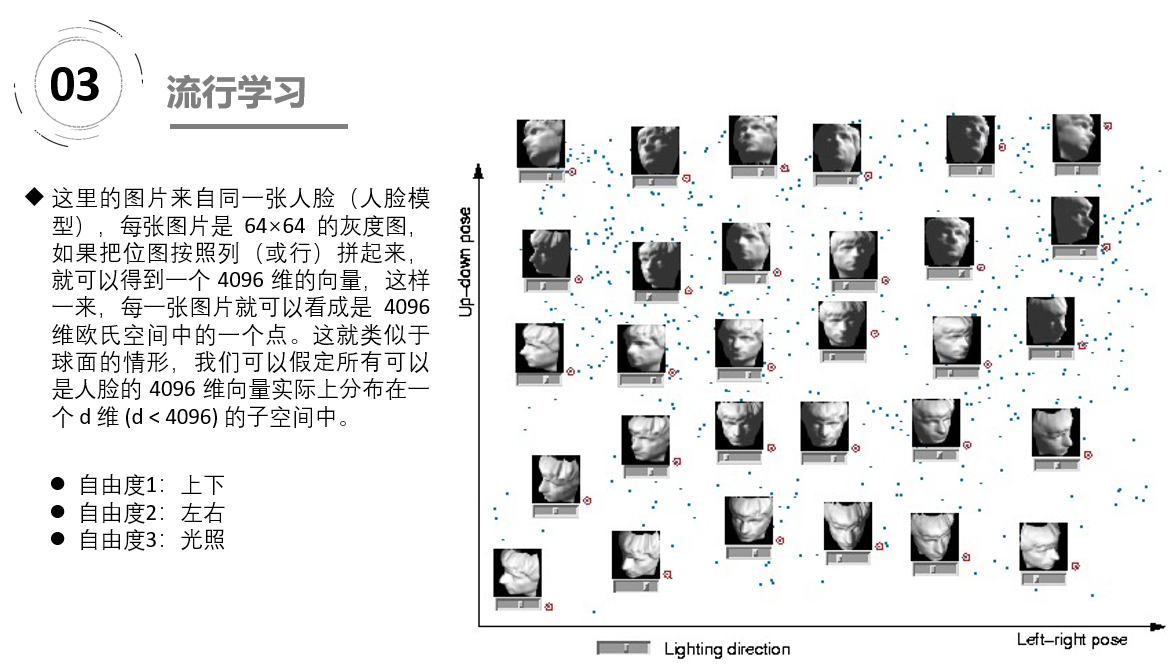不过是在不同的 pose 和光照下拍摄的，如果把 pose （上下和左右）当作两个自由度，而光照当作一个自由度，那么这些图片实际只有三个自由度，换句话说，存在一个类似于球面一样的参数方程（当然，解析式是没法写出来的），给定一组参数（也就是上下、左右的 pose 和光照这三个值），就可以生成出对应的 4096 维的坐标来。换句话说，这是一个嵌入在 4096 维欧氏空间中的一个 3 维流形。

展开全文• 文章目录表示学习特征工程与表示学习深度学习的表示学习注：深度学习不等于多层神经网络什么时候用「手工提取」什么时候用「表示学习」？关于特征表示学习的算法参考资料 表示学习 表示学习的基本思路，是找到对于...
文章目录表示学习特征工程与表示学习深度学习的表示学习注：深度学习不等于多层神经网络什么时候用「手工提取」什么时候用「表示学习」？关于特征表示学习的算法参考资料
表示学习
表示学习的基本思路，是找到对于原始数据更好的表达，以方便后续任务（比如分类）。机器学习中，同样的数据的不同表达，会直接决定后续任务的难易程度（换言之，表示方法的选择通常依赖于后续任务的需求），因此找到好的数据表示往往是机器学习的核心任务。
特征工程与表示学习
机器学习一般有两种思路来提升原始数据的表达：

特征学习(feature learning)，又叫表示学习(representation learning)或者表征学习，一般指模型自动从数据中抽取特征或者表示的方法，是模型自动学习的过程；
特征工程(feature engineering)，主要指对于数据的人为处理提取，得到我们认为的适合后续模型使用的样式，是人工提取的工程 （狭义的特征工程指的是“洗数据”：处理缺失值，特征选择，维度压缩等各种预处理手段，但从更广义的角度看，这些处理是为了使得数据有更好的表达以便后续应用）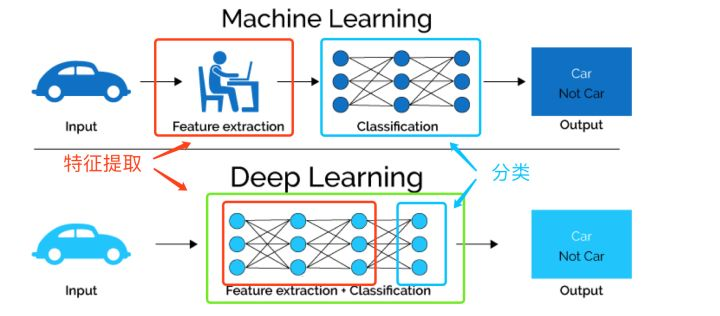传统的机器学习方法主要依赖人工特征处理与提取，而深度学习则依赖模型自身去学习数据的表示（自动抽取有效特征）。
深度学习的表示学习
输入数据经过层层网络，依次被抽取出了低级特征（low level features）比如边缘色度，中级特征（middle level features）比如纹理角点，和高级特征比如图形，然后把高度抽象化的高级特征交给最后的分类器层进行预测，从而得到分类结果。深度网络最后一层一般就是个线性分类器，比如softmax线性回归分类，深度神经网络的其他部分可以看做是为最后一层的分类器提供表征。通过层层网络抽取高度抽象化的特征，最终目的是为了帮助分类器做出良好的预测：最开始输入网络的特征可能是线性不可分的，但是到最后隐藏层时变得线性可分了。如果只看分类器层的话深度学习和其他机器学习没有太大差别，正是前面层层网络良好有效的抽取特征的能力使得深度学习脱颖而出 。
注：深度学习不等于多层神经网络
传统意义上的多层神经网络只有输入层，隐藏层，输出层。其中隐藏层的层数根据需要而定。“深度学习的网络结构”广义上就属于多层神经网络，只是更深更复杂。而"深度学习"是为了让层数较多的多层神经网络可以训练、能够work而演化出来的一系列的 新的结构和新的方法 。
新的网络结构中最著名的就是CNN，它在多层神经网络的基础上，加入了“卷积层“进行特征学习，符合人脑对视觉任务的处理方式；并且其“局部感受野”和“权植共享”的概念，大大减少了网络参数的数量，解决了传统较深的网络参数太多难以训练的问题。新的结构还包括了：LSTM，ResNet等。
新的方法就多了：新的激活函数（ReLU），新的权重初始化方法（逐层初始化，XAVIER等），新的损失函数，新的防止过拟合方法（Dropout, BN等）。这些方面主要都是为了解决传统的多层神经网络的一些不足：梯度消失，过拟合等。
什么时候用「手工提取」什么时候用「表示学习」？
一般来说，要想构造良好的特征工程，需要深刻理解数据和问题；要想自动学习到数据的良好表达，就需要大量的数据。因此选择的过程其实也是在衡量我们对于问题及数据的理解是否深刻，是人类先验与数据量之间的一场博弈。一般来说，「特征工程」往往在中小数据集上表现良好，而「表示学习」在大量复杂数据上更有用武之地。当数据量不大且对数据充分理解时，特征工程比较合适，这在kaggle竞赛中很常见。而当数据量较大或者我们的先验理解有限时，可尝试表示学习，比如神经网络。另外，表示学习的另外一个好处是高度抽象化的特征可以通过迁移学习用在其他相关的问题上 。不管是特征工程还是表示学习，我们衡量它们作用的一个标准就是看后续的学习任务是否能够得益于这种表示。
关于特征给出一张猫的图片，我们的大脑是怎么识别出这是一只猫的呢？通过眼睛，耳朵，嘴巴，尾巴，腿，或是这些的组合？这里的“耳朵，嘴巴，尾巴" 等等就是我们用来判断的"特征"，大脑的神经元迅速完成了一系列复杂的运算，最后得出结论这是猫。再比如“四条边，等长，直角”这些稍低级的特征可被大脑用来识别正方形。
但对于计算机而言，图片只是一堆数字而已，比如800600的彩色图片就是一个8006003的矩阵（灰度图则为800600的矩阵），矩阵里相应元素的值就代表着像素值（像素值也是人为创造的概念，对计算机来说这就只是个数字）。那么什么样的数字代表“猫的眼睛”，什么样的数字代表“猫的耳朵”呢？如果采用特征工程，就需要理解数据并且人为定义规则来提取特征，比如定义连续5个像素（1,34,67,89,213）则代表猫尾，… ，当然定义的规则，和提取特征的好坏，非常影响最终的分类结果 。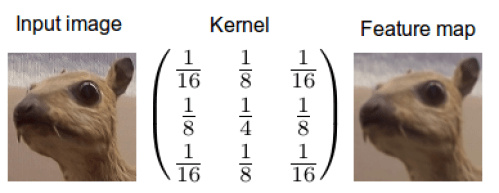我们可以利用像素和邻域像素之间的差异，设计卷积核来提取图像的局部特征。经过不同卷积核的卷积运算后，可以起到不同的作用，比如高斯平滑卷积核可以被看做每个像素被其邻居像素平均（边缘模糊），见上图；而边缘检测的卷积核，就是将每个像素和其邻域像素做差值，如下图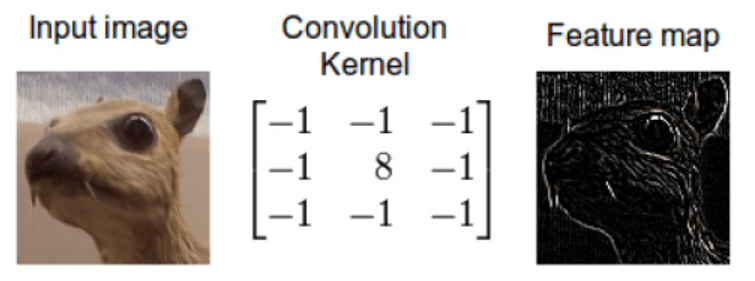再比如识别一个曲线，可以按照曲线的形状走向设计卷积核的形状，在遇到类似图像的时候，卷积运算后的数值会很大（对应位置像素值相乘后相加），反之很小，即可识别想要的形状。
注：卷积运算其实就是向量的内积运算。内积是衡量两个向量之间的相似性的，所以卷积核在图像某一区域的卷积实际上就是这个卷积核与图像该区域的相似性。一个卷积核探索一种相似性，多个卷积核探索多个相似性。卷积核作用在同一样本的不同位置上（即卷积核在整张图上共享：权值共享）那么kernel探索的就是不同位置共有的局部特征，即局部特征的平移不变性。卷积核作用在不同的样本上，那么kernel 探索的是样本数据共有的局部特征。
以上也是卷积核又称为滤波器或者模板的原因 。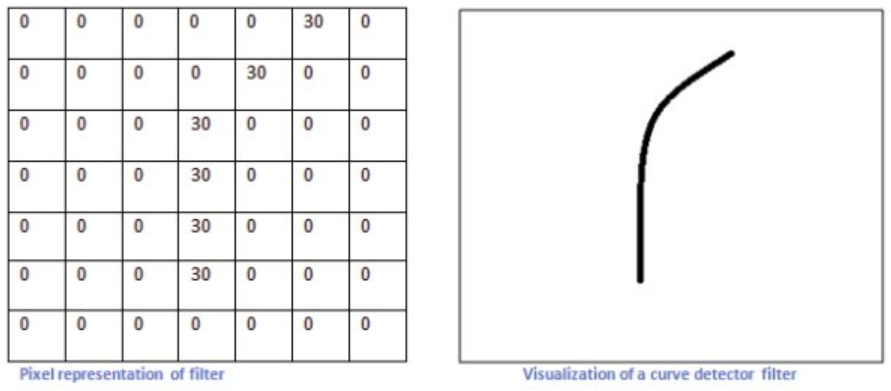传统的图像处理，就是人工设计好了不同的卷积核（滤波器）去提取不同特征，常见的滤波器：高通、低通、高斯模糊、SOBEL 查找边缘 … 是白盒。但非常依赖经验，提取规则只适用特定数据和问题，对于某些任务，特征并不单一和具体，很难设计适合的滤波器。比如计算机视觉领域的目标检测：想要设计一个卷积核检测眼睛位置，但是不同的人，眼睛大小状态是不同的，如果卷积核太过具体化，卷积核代表一个睁开的眼睛特征，给出一张图片的眼睛是闭合的，就很大可能检测不出来。对于这种问题，我们如何设计卷积核呢，即，如何确定卷积核的值呢？
深度学习可以自动寻找合适的卷积核来完成特征提取（卷积核的固定值被替换为参数来求解），得到的“滤波器”是黑盒，神经网络不需要理解数字代表的业务含义，它只需要尝试找到最合适的卷积核等各种参数，使得在给定数据上loss最小就可以了 （求解卷积核参数的过程涉及到损失函数，梯度下降，反向传播等：使得最终的卷积核，通过它提取出来的特征，能够使预测得到的结果和真值尽可能接近）。
当然了，虽然深度学习的过程不要人为理解数字背后的业务含义，但实际上深度学习得到的特征是具有一定语义的：学习到的低级特征可视化后得到一些点，线，方向，颜色等等，中级特征可视化后可能是一些轮廓形状，角点等等，高级特征就更加抽象了甚至有时人类并不能理解。这也是迁移学习和fine-tune存在的基础，一个经过大型图像数据集如ImageNet训练过的大规模CNN，因为前面几层已经通过训练得到了丰富的底层细节信息，所以只需要在新数据集上训练上层网络（对低级特征的组合信息）以及最后的分类器层即可，完全不需要从头再来 。
小结，深度学习并不“理解”所识别物体的外观/纹理/颜色等“特征”… 它只是能够根据这些本质仍为数字的“特征”，进行分类/分割/… 任务。它们所做的实际就是两件事情：将（输入）视觉空间分解为卷积滤波器的分层模块化网络（类似于人类视觉皮层系统）；将这些滤波器的某些组合与给定标签之间建立概率映射 。
表示学习的算法
大致包括：

深度学习，包括大部分常见的模型如CNN/RNN/DBN/GCN等
某些无监督学习算法，如主成分分析(PCA)及自编码器（autoencoder）通过对数据转化而使得输入数据更有意义
某些树模型可以自动的学习到数据中的特征并同时作出预测

深度神经网络的最后一层一般都是一个线性分类器，其他层则可看成是在给这个线性分类器提供特征，所以最后一层之前的隐藏层都可被理解为是表征学习，在上一节中有更具体的描述。
在深度学习大幅发展之前，无监督学习是很好的表征学习的工具，精髓是学习从“高维观察” 到 “低维表征空间” 的映射，使得可通过低维的表征（近似地）重建出原来的观察，比如PCA的映射空间就保证了原空间的多样性。自编码器是一种无监督的神经网络，它与PCA很相似，需要最小化和PCA一样的目标函数，但相比于PCA只能执行线性变换，自动编码器既能表征线性变换，也能表征非线性变换。因为自动编码器的网络表征形式，所以可将其作为层用于构建深度学习网络。
参考资料
「特征工程」与「表示学习」 - 微调的文章 - 知乎
 深度学习 和 多层神经网络 的区别? - 知乎
 深度学习的一些思考
 cnn本质
 How convolutional neural networks see the world – The Keras Blog
 自动编码器


展开全文• ## 知识表示学习模型

万次阅读 2019-10-01 13:43:34
写在前面 最近清华的THUNLP整理了Pre...本来这篇是打算写一写Knowledge Graph + BERT系列工作的，但是最近有在做知识图谱的一些东西所以就先整理一下知识表示学习(knowledge representation learning)的相关模型发展...
最近清华的THUNLP整理了Pre-trained Languge Model (PLM)相关的工作：PLMpapers，非常全面，想要了解最新NLP发展的同学不要错过。本来这篇是打算写一写Knowledge Graph + BERT系列工作的，但是最近有在做知识图谱的一些东西所以就先整理一下**知识表示学习(knowledge representation learning)**的相关模型发展，这样我们看后面的paper也会得心应手一些。1. A glance at Knowledge Representation Learning
What
首先什么是表示学习？其实我们早就在频繁使用了，比如词向量embedding，这种将研究对象的语义信息表示为稠密低维的实值向量的形式就叫表示学习。
有了表示学习的定义，那么知识表示学习(KRL) 就是面向知识库中实体和关系的表示学习，通过将实体或关系投影到低维向量空间，能够实现对实体和关系的语义信息的表示，可以高效地计算实体、关系及其之间的复杂语义关联。
Why
那么为什么会冒出知识表示学习呢？

显著提高计算效率。 简单地基于图算法计算实体间的语义和推理关系，其计算复杂度高、可扩展性差；而表示学习得到的分布式表示，则能够高效地实现语义相似度计算等操作。
有效缓解数据稀疏。 一方面将每个对象投影到低维的稠密向量空间；另一方面在投影过程中也可以借用高频对象的语义信息帮助低频对象的语义表示，捕获长尾分布。
实现异质信息融合。 将不同来源的对象投影到同一语义空间中，就能够建立统一的表示空间。

How
接下去会介绍知识表示学习的主要方法及前沿进展，这也是本系列博文的重要。首先给出整体定义：

知识库表示为 G=(E, R, S)，其中$E={\{e_{1},e_{2}, \cdots, e_{E}\}}$是实体集合，$R=\left\{r_{1}, r_{2}, \cdots, r_{R}\right\}$是关系集合，S是三元组集合，表示为(h, r, t)

基于距离的模型
基于翻译的模型
语义匹配模型
融合多源信息的模型
前沿进展

paper list ref

清华Must-read papers on KRL/KE
东南大学《知识图谱》课程

2. Distance-Based Models
UM Joint Learning of Words and Meaning Representations for Open-Text Semantic Parsing(2012)SELearning Structured Embeddings of Knowledge Bases(AAAI/2011)
在本文中，作者指出目前已有的知识库都是基于不同的框架，因此很难将它们整合应用到一个新的系统中。但是知识库中存在大量的结构化和组织化的数据，如果能够充分利用起来将会对AI领域非常有帮助。于是作者们提出一种模型将任何知识库中的实体和关系嵌入到一个更灵活的连续向量空间。嵌入可以认为是一个神经网络，该神经网络的特殊结构允许将原始数据结构集成到所学习的表示中。更准确地说，考虑到知识库是由一组实体和它们之间的关系定义的，该模型为每个实体（即一个低维向量）学习一个嵌入(embedding)，为每个关系（即矩阵）学习一个运算符(operator)。另外，在低维embedding空间使用核密度估计可以估量空间的概率密度，这样可以量化实体之间的关系存在可能性。
结构表示（Structured Embeddings，SE）中每个实体用$d$维的向量表示，所有实体被投影到同一个$d$维向量空间中。同时，SE还为每个关系定义了两个矩阵$\boldsymbol{M}_{r, 1}, \boldsymbol{M}_{r, 2} \in \mathbb{R}^{d \times d}$用于三元组中头实体和尾实体的投影操作。最后SE为每个三元组$(h, r, t)$定义了损失函数：
$f_{r}(h, t)=\left|\boldsymbol{M}_{r, 1} \boldsymbol{l}_{h}-\boldsymbol{M}_{r, 2} \boldsymbol{l}_{t}\right|_{L_{1}}$对于上述损失函数，我们可以理解为通过两个关系矩阵将头尾两个实体投影到同一关系空间中，然后在该空间中计算两投影向量的距离。而这个距离则反映了头尾实体之间在特定关系下的语义相似度，他们的距离越小则表明越有可能存在这种关系。
SE模型要学习的参数是实体向量矩阵$E$ 和两个关系矩阵$R^{lhs}$、$R^{rhs}$，下面是模型的训练过程：可以看出SE模型对头尾两个实体使用不同的矩阵进行投影，协同性较差，往往无法精确刻画两实体之间的语义联系。

3.  Trans-Based Models
TransE Translating Embeddings for Modeling Multi-relational Data(NIPS2013)
TransE是Trans系列模型的开山之作，也是知识图谱向量表示的baseline模型，后面会介绍它的很多变体。其实TransE的思想很简单，为了将实体与关系嵌入到低维的向量空间，对于知识库中的每个三元组$(h, r, t)$，认为头实体的embedding加上关系的embedding之后应该是与尾实体的embedding非常接近的。于是，可以将关系看做是两个实体之间的某种平移向量，如下图：按照上面的思路，即有了TransE模型的损失函数：$\mathcal{L}=\sum_{(h, \ell, t) \in S\left(h^{\prime}, \ell, t^{\prime}\right) \in S_{(h, \ell, t)}^{\prime}}\left[\gamma+d(\boldsymbol{h}+\ell, \boldsymbol{t})-d\left(\boldsymbol{h}^{\prime}+\ell, \boldsymbol{t}^{\prime}\right)\right]_{+}$这个损失函数是带有negative sampling和margin-based loss的

negative sampling：$S_{(h, \ell, t)}^{\prime}=\left\{\left(h^{\prime}, \ell, t\right) | h^{\prime} \in E\right\} \cup\left\{\left(h, \ell, t^{\prime}\right) | t^{\prime} \in E\right\}$表示负样本三元组，构造的方法是将S中每个三元组的头实体、关系和尾实体其中之一随机替换成其他的实体或关系，注意每个三元组只能同时替换一个元素。
margin-based loss：为了增强知识表示的区分能力，采用了最大间隔的方法，可以类比SVR的损失函数。$d(\boldsymbol{h}+\ell, \boldsymbol{t})$表示正样本的不相似得分，$d\left(\boldsymbol{h}^{\prime}+\ell, \boldsymbol{t}^{\prime}\right)$表示负样本的不相识得分，最小化这个损失函数可以使得正样本的得分越来越高而负样本的得分越来越低，并且只有两个得分差距大于某一阈值的时候loss才为0。

最终模型整体的训练过程如下：虽然TransE简单有效，但仍然存在一个非常严重的问题：不能很好处理复杂关系。举个栗子，有两个三元组(美国，总统，奥巴马)和(美国， 总统， 布什)，使用TransE模型表示的话会得到奥巴马和布什的表示向量非常接近，甚至完全相同。Code Here

TransHKnowledge Graph Embedding by Translating on Hyperplanes(AAAI2014)
相对于之前的知识表示模型，TransE有了很大的改进，但是当面对自反关系、一对多、多对一以及多对多这类复杂的关系时，就很难处理好（会使得一些不同的实体具有相同或者相近的向量表示）。于是作者提出TransH模型，在保证模型complexity和efficiency的同时，解决上述复杂关系的表示。
模型
TransH模型的主要思想是对每一个关系定义两个向量：超平面$w_{r}$和关系向量$d_{r}$。对于任意一个三元组$(h, r, t)$，$h$和$t$在超平面上的投影分别为$h_{\perp}$和$t_{\perp}$，如果是golden triplet，则存在$h_{\perp}+d_{r} \approx t_{\perp}$。看下图可能会更清楚一些：损失函数与TransE的一样：$\mathcal{L}=\sum_{(h, r, t) \in \Delta\left(h^{\prime}, r^{\prime}, t^{\prime}\right) \in \Delta_{(h, r, t)}^{\prime}}\left[f_{r}(\mathbf{h}, \mathbf{t})+\gamma-f_{r^{\prime}}\left(\mathbf{h}^{\prime}, \mathbf{t}^{\prime}\right)\right]_{+}$$f_{r}(\mathbf{h}, \mathbf{t})=\left\|\left(\mathbf{h}-\mathbf{w}_{r}^{\top} \mathbf{h} \mathbf{w}_{r}\right)+\mathbf{d}_{r}-\left(\mathbf{t}-\mathbf{w}_{r}^{\top} \mathbf{t} \mathbf{w}_{r}\right)\right\|_{2}^{2}$$\mathbf{h}_{\perp}=\mathbf{h}-\mathbf{w}_{r}^{\top} \mathbf{h} \mathbf{w}_{r}, \quad \mathbf{t}_{\perp}=\mathbf{t}-\mathbf{w}_{r}^{\top} \mathbf{t} \mathbf{w}_{r}$
模型整体训练过程也同TransE一样，这里就不再赘述。
负采样策略
不同于TransE模型中的随机替换头实体和尾实体之一作为负样本的方法，在TransH中指出：

However, as a real knowledge graph is often far from completed, this way of randomly sampling may introduce many false negative labels into training.

于是，在替换头实体或者尾实体的时候考虑了一个采样概率，$\frac{t p h}{t p h+h p t}$的概率替换头实体，$\frac{h p t}{t p h+h p t}$的概率替换尾实体，其中$tph$为每个头实体对应尾实体的平均数量，$hpt$为每个尾实体对应头实体的平均数量。也就是说，对于一对多的关系，更大的概率替换头实体；对于多对一的关系，更大概率替换尾实体。
Code Here

TransR Learning Entity and Relation Embeddings for Knowledge Graph Completion(AAAI2015)
前面介绍的TransE和TransH都是假设三元组的实体和关系在同一语义空间中的，尽管在TransH中采用了关系超平面的策略，但由于实体和关系是完全不同的，每一个实体可能会有多个aspect，即是是同一个实体在不同的关系下关注的可能是完全不相关的aspect，因此之前的模型还是不足以准确地表示knowledge graph。
于是，在本文中作者提出一种新模型TransR，其思想就是将实体和关系的表示空间区分开来，实体有entity space，关系有relation space，在计算时通过一个投射矩阵$M_{r}$将实体映射到关系空间进行计算。
$投影过程：\mathbf{h}_{r}=\mathbf{h} \mathbf{M}_{r}, \quad \mathbf{t}_{r}=\mathbf{t} \mathbf{M}_{r}$$打分函数： f_{r}(h, t)=\left\|\mathbf{h}_{r}+\mathbf{r}-\mathbf{t}_{r}\right\|_{2}^{2}$$损失函数：L=\sum_{(h, r, t) \in S\left(h^{\prime}, r, t^{\prime}\right) \in S^{\prime}} \max \left(0, f_{r}(h, t)+\gamma-f_{r}\left(h^{\prime}, t^{\prime}\right)\right)$此外，不仅仅实体有多个aspect，对于关系，也可能存在多义性，比如关系（location location contains）其实包含country-city、country-university、continent-country等多种含义。为此，作者们提出CTransR，即Cluster-based TransR，对同一关系下的不同实体对进行聚类并学习到关系在不同聚类簇的表示。具体做法是

利用TransE训练$(h, r, t)$三元组
利用vector offset $(h-t)$进行聚类
对每一类的子关系都学习到一个变换矩阵$M_{r}$和表示向量$r_{c}$
$f_{r}(h, t)=\left\|\mathbf{h}_{r, c}+\mathbf{r}_{c}-\mathbf{t}_{r, c}\right\|_{2}^{2}+\alpha\left\|\mathbf{r}_{c}-\mathbf{r}\right\|_{2}^{2}$

Code Here

TransDKnowledge graph embedding via dynamic mapping matrix(ACL2015)
文章首先指出了TransR的三个问题：

在TransR中，对于特定关系，所有实体共享同一映射矩阵。但是实际情况头尾实体可能属于不同范畴，例如(美国，总统，特朗普)这个三元组中，美国和特朗普就不是相同范畴的实体，因此应该采用不同的映射矩阵；
投影运算是实体与关系的交互过程，映射矩阵仅由关系确定是不合理的；
矩阵向量乘法运算量大，当关系数大时，矩阵向量乘法的参数也比TransE和TransH多。

基于此，作者们提出一种基于动态映射矩阵的TransD模型。对于每个三元组$(h, r, t)$，其中每个元素都有两种向量表示，一种是构建动态映射矩阵向量$(h_{p}, r_{p}, t_{p})$；另外一种是用于自身实体/关系语义表示$(\bold h, \bold r, \bold t)$。首先是利用头尾实体的映射向量构建映射矩阵：\begin{aligned} \mathbf{M}_{r h} &=\mathbf{r}_{p} \mathbf{h}_{p}^{\top}+\mathbf{I}^{m \times n} \\ \mathbf{M}_{r t} &=\mathbf{r}_{p} \mathbf{t}_{p}^{\top}+\mathbf{I}^{m \times n} \end{aligned}接着通过各自的映射矩阵将实体投影到关系空间：$\mathbf{h}_{\perp}=\mathbf{M}_{r h} \mathbf{h}, \quad \mathbf{t}_{\perp}=\mathbf{M}_{r t} \mathbf{t}$整体的打分函数和损失函数以及训练过程都跟之前的模型一样。Code Here

TranSparse Knowledge Graph Completion with Adaptive Sparse Transfer Matrix(AAAI2016)
之前的Trans系列模型都忽略了知识表示面临的两个问题：

异构性(heterogeneous)：不同关系连接的实体对数量差别巨大
不平衡性(imbalanced)：同一关系链接的头实体和尾实体数量差别巨大，例如对于关系性别，头实体的数量很多（‘小明’‘小花’etc），而对于尾实体仅仅只有两个（‘男’‘女’）如果使用同一模型来处理所有情况的话，则可能由于异构性导致对于复杂关系（链接数量多）欠拟合，对简单关系（链接数量少）过拟合。为了解决异构性，作者提出TranSparse模型用自适应的稀疏矩阵代替一般的映射矩阵。
TranSparse(share)
为了解决异构性问题，TranSparse将transfer matrix设置为自适应的稀疏矩阵，对于复杂关系，我们需要更多的参数去学习其中包含的信息，所以transfer matrix的稀疏度会比较低，即有更多的元素不为0；而对于简单的关系则恰好相反。而这里稀疏度由$\theta_{r}$定义：
$\theta_{r}=1-\left(1-\theta_{\min }\right) N_{r} / N_{r^{*}}$其中，$\theta_{min}$是一个0-1之间的最小稀疏度超参，$N_{r}$表示关系$r$链接的实体对数量，$N_{r^{*}}$表示其中链接的最大值。
TranSparse(share)这里没有考虑不平衡性，所以对于头尾实体的mapping过程是一样的：$\mathbf{h}_{p}=\mathbf{M}_{r}\left(\theta_{r}\right) \mathbf{h}, \quad \mathbf{t}_{p}=\mathbf{M}_{r}\left(\theta_{r}\right) \mathbf{t}$
TranSparse(separate)
为了解决不平衡性问题，这里更细致地对头实体和尾实体设置了不同的transfer matrix：$\theta_{r}^{l}=1-\left(1-\theta_{\min }\right) N_{r}^{l} / N_{r^{*}}^{l^{*}} \quad(l=h, t)$$\mathbf{h}_{p}=\mathbf{M}_{r}^{h}\left(\theta_{r}^{h}\right) \mathbf{h}, \quad \mathbf{t}_{p}=\mathbf{M}_{r}^{t}\left(\theta_{r}^{t}\right) \mathbf{t}$
模型训练
两者（share和separate）的打分函数都是：$f_{r}(\mathbf{h}, \mathbf{t})=\left\|\mathbf{h}_{p}+\mathbf{r}-\mathbf{t}_{p}\right\|_{\ell_{1 / 2}}^{2}$$L=\sum_{(h, r, t) \in \Delta\left(h^{\prime}, r, t\right) \in \Delta^{\prime}}\left[\gamma+f_{r}(\mathbf{h}, \mathbf{t})-f_{r}\left(\mathbf{h}^{\prime}, \mathbf{t}^{\prime}\right)\right]_{+}$Code Here

TransM Transition-based knowledge graph embedding with relational mapping properties(2014)
TransM也是为了解决TransE只能处理一对一关系的问题，对于存在多种选择的一方给了更多的自由度，即$h+r \approx t$，如下图具体做法是在得分函数的前面加上了权重$w_{r}$
$w_{r}=\frac{1}{\log \left(h_{r} p t_{r}+t_{r} p h_{r}\right)}$其中$h_{r}pt_{r}$表示尾实体对应的头实体数量(heads per tail)，$t_{r}ph_{r}$同理。$打分函数： f_{r}(h, t)=w_{\mathbf{r}}\|\mathbf{h}+\mathbf{r}-\mathbf{t}\|_{L_{1} / L_{2}}$

ManiFoldE From One Point to A Manifold: Knowledge Graph Embedding For Precise Link Prediction(IJCAI2016)
作者指出目前已有的研究都无法很好地解决精准链接预测(Precise Link Prediction)，主要是有两个问题：

ill-posed algebraic problem：指一个方程组中的方程式个数远大于变量个数，这会导致求得的解经常是不精确且不稳定的。之前的翻译模型都是基于$\mathbf{h}_{\mathbf{r}}+\mathbf{r}=\mathbf{t}_{\mathbf{r}}$，如果三元组的数量为$T$，embedding的维度为$d$， 则一共有$T*d$个方程，而所需要学习的变量一共有$(E+R)*D$个，由于$T>>(E+R)$，那么这类模型由于ill-posed algebraic problem无法给出精准链接预测；
adopting an overstrict geometric form：TransE过于严格的限制使得链接预测得到的基本都为一个点，这对于多对多关系显然是不正确的。虽然在之前有TransH， TransR等模型对此进行优化，但是将关系映射到另外一个子空间后仍然会存在该问题。

为了解决上述两个问题，作者提出一种基于流形的模型，将约束$\mathbf{h}_{\mathbf{r}}+\mathbf{r}=\mathbf{t}_{\mathbf{r}}$进行放宽$\mathcal{M}(\mathbf{h}, \mathbf{r}, \mathbf{t})=D_{r}^{2}$其中$\mathcal{M}$是流形函数$M(h, r, t)=\|h+r-t\|_{l 2}$
以$(h, r, *)$为例，所有合适的尾实体都分布在高维流形上，举个栗子，$\mathcal{M}$是一个高维球体，则所有的尾实体都在以$h+t$为球心，以$D_{r}$为半径的球面上。
$打分函数：f(h, r, t)=\left\|M(h, r, t)-D_{r}^{2}\right\|_{l 1 / 2}$
$目标函数：\mathcal{L}=\sum_{(h, r, t)} \sum_{\left(h^{\prime}, r^{\prime}, t^{\prime}\right) \in \Delta^{\prime}}\left[f_{r}^{\prime}\left(h^{\prime}, t^{\prime}\right)-f_{r}(h, t)+\gamma\right]_{+}$
Code Here

TransF Knowledge Graph Embedding by Flexible Translation(2016)
TransF提出主要是为了解决TransE的一对一关系问题，虽然在之前有TransH提出解决思路，但是引入了更多的参数以及更大的复杂度。于是TransF就是为了解决一对多多对一以及多对多关系，同时又不增加参数。具体就是将TransE的限制条件$\mathbf{h}+\mathbf{r} \approx \mathbf{t}$放宽为$\mathbf{h}+\mathbf{r} \approx \alpha \mathbf{t}$，如下图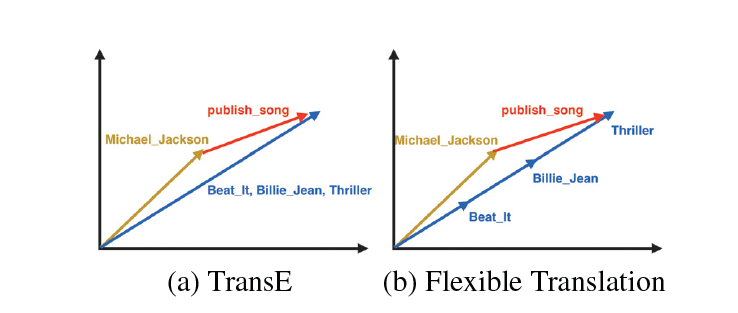得分函数同时衡量了$h+t$和$t$，$t-h$和$h$的方向：
$f(h, r, t)=(h+r)^{T} t+(t-r)^{T} h$

TransA TransA: An Adaptive Approach for Knowledge Graph Embedding(2015)
作者首先提出了两个TransE模型的不足：

loss metric：TransE的loss metric为$\|\mathbf{h}+\mathbf{r}-\mathbf{t}\|_{2}^{2}$，是一种欧氏距离的计算，其对应的图形是一个圆。由于这种简单的loss metric导致不能很好处理复杂的关系（如一对多、多对一等）。论文中给出一个详细案例，从下图可知蓝色部分为正例，红色部分为负例，TransE 模型划分错了七个点。而本文提出了一种基于马氏距离的 TransA 模型，其 PCA 降维图形对应的是一个椭圆，该模型只分错了三个点。identical dimension：TransE等之前的模型等价地对待向量中的每一维度，但是实际上各个维度的重要度是不相同的，有些维度是有作用的，有些维度却是噪音。还是以论文中的栗子，对于关系HasPart来说，左边的TransE模型根据欧氏距离计算得出(room, haspart, goniff)这样错误的三元组。通过对x，y轴进行分解之后发现，关系HasPart会更关注与x轴相近的实体。TransA模型为此引入了对每一维度的加权矩阵。模型
TransA模型对之前的打分函数进行了改进，引入加权矩阵$W_{r}$：$f_{r}(h, t)=(|\mathbf{h}+\mathbf{r}-\mathbf{t}|)^{\top} \mathbf{W}_{\mathbf{r}}(|\mathbf{h}+\mathbf{r}-\mathbf{t}|)$并通过LDL对$W_{r}$进行分解：$\mathbf{W}_{\mathbf{r}}=\mathbf{L}_{\mathbf{r}}^{\top} \mathbf{D}_{\mathbf{r}} \mathbf{L}_{\mathbf{r}}$整理后得到最终的打分函数：$f_{r}=\left(\mathbf{L}_{\mathbf{r}}|\mathbf{h}+\mathbf{r}-\mathbf{t}|\right)^{\top} \mathbf{D}_{\mathbf{r}}\left(\mathbf{L}_{\mathbf{r}}|\mathbf{h}+\mathbf{r}-\mathbf{t}|\right)$训练过程损失函数\begin{aligned} \min & \sum_{(h, r, t) \in \Delta\left(h^{\prime}, r^{\prime}, t^{\prime}\right) \in \Delta^{\prime}}\left[f_{r}(h, t)+\gamma-f_{r^{\prime}}\left(h^{\prime}, t^{\prime}\right)\right]_{+}+\\ & \lambda\left(\sum_{r \in R}\left\|\mathbf{W}_{\mathbf{r}}\right\|_{F}^{2}\right)+C\left(\sum_{e \in E}\|\mathbf{e}\|_{2}^{2}+\sum_{r \in R}\|\mathbf{r}\|_{2}^{2}\right) \\ \text { s.t. } &\left[\mathbf{W}_{\mathbf{r}}\right]_{i j} \geq 0 \end{aligned}

KG2E Learning to Represent Knowledge Graphs with Gaussian Embedding(2015)
作者指出TransE、TransR等之前的研究，在分离正三元组及其对应的负三元组时，不同的实体和关系往往共享相同的边界，而知识库中实体和关系的**（非）确定性**被完全忽略。但实际上，不同的实体和关系常常包含不同的确定性。在这里，一个实体/关系的（非）确定性表示在将一个三元组作为上下文评分时表示其语义的可信度。举个栗子，在推断一个人时，配偶关系的确定性明显大于国籍。在预测Hillary Clinton时，当我们知道她丈夫（配偶）是Bill Clinton时，我们可能更有信心知道她是谁，而不是知道她出生在（国籍）美国。
为此，本文使用Gaussian Distribution 来表示实体和关系，使用协方差来表示实体和关系的不确定度。相同颜色的圆圈代表同意三元组，圆圈大小则表示不同三元组的不确定度。相对于之前的point-based model，本文的KG2E是一种density-based model。在建模过程中强调了(un)certainty的概念，融入关系和实体语义本身的不确定性并使用高斯分布协方差表示实体关系的不确定度，使用高斯分布的均值表示实体或关系在语义空间中的中心值。
但是KG2E在link prediction任务中的多对多关系上表现不是很好，主要原因是模型没有考虑实体和关系的类型和粒度。

TransG TransG : A Generative Model for Knowledge Graph Embedding(2016)
本文主要是为了解决多关系语义（multiple relation semantics），即某一种关系可能会存在多个类别的语义，比如对于(Atlantics, HasPart, NewYorkBay)和(Table, HasPart, Leg)两个三元组都属于关系HasPart，但是他们在语义上并不相同，一个是位置的语义，一个是部件的语义。其实这个问题在之前介绍的CTransR中也提出过解决方案，但是作者似乎认为CTransR预处理聚类的操作不是很elegant。为此，作者们提出了一种基于贝叶斯非参的无限混和嵌入模型：认为关系向量由若干子成分向量合成，模型会根据实体对自动选择一个关系向量，而多少个这样的关系向量还可以由模型自动选择。 整体过程如下：一张总结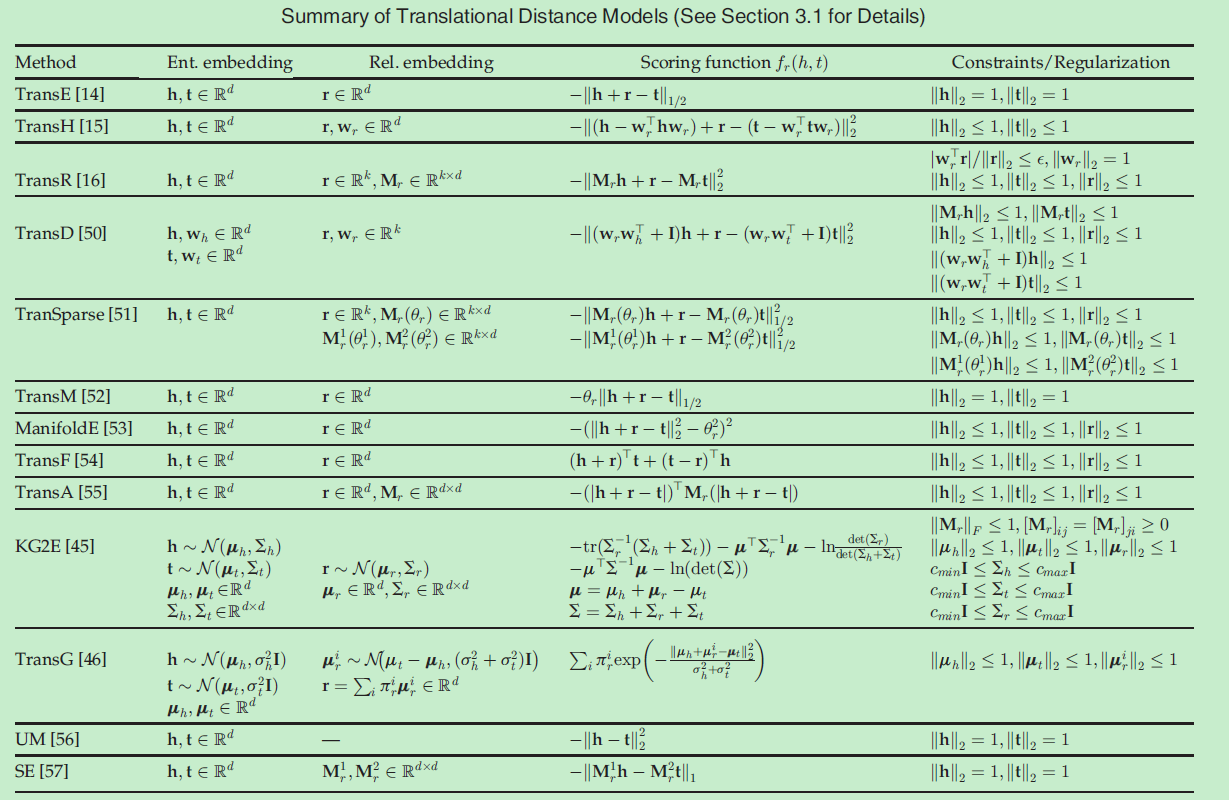以上
2019.10.08


展开全文Trans系列
• ## 网络表示学习总结

万次阅读 2017-07-03 10:47:27
最近看了paperweekly的两次关于网络表示学习的直播，涂存超博士与杨成博士讲解了网络表示学习的相关知识。本文将网络表示学习中的一些基本知识，结合自己的一些粗浅的理解，整理记录下来。
• 深度学习是表示学习的经典代表： 深度学习的过程： 深度学习与传统机器学习差别： 深度学习代表算法： 先来看机器学习： 机器学习是利用经验experience来改善 计算机系统自身的性能，通过经验获取知识knowledge...
• 本章的表示学习主要就是通过无监督训练学习特征的意思。 这种没有具体的算法，就是介绍表示学习的应用和大概的分支，至于如何进行表示学习，没有详细介绍。感觉可以直接跳过。。 贪心逐层无监督预训练 贪心算法在...
• ## 知识图谱表示学习

千次阅读 2018-06-26 10:49:03
知识图谱是一种精细化的异构网络, 所以对其节点与边的表示学习也是一个热门的问题. 这里的学习依旧是得到它们的低维稠密向量. 参考 论文transr
• Paper: 刘知远 2016 知识表示学习研究进展 计算机研究与发展 1. 表示学习旨在将研究对象的语义信息表示为稠密低维表示向量；而知识表示学习则面向知识库中的实体和关系进行表示学习；该技术可以在低维空间中高效...知识图谱 TransR
• ## 网络表示学习笔记

千次阅读 2018-04-12 19:57:09
网络表示学习（Representation Learning on Network），一般说的就是向量化（Embedding）技术，简单来说，就是将网络中的结构（节点、边或者子图），通过一系列过程，变成一个多维向量，通过这样一层转化，能够将...
• DeepNLP的表示学习·词嵌入来龙去脉·深度学习（Deep Learning）·自然语言处理（NLP）·表示（Representation） 词向量、word embedding、分布式表示、word2vec、glove 术语解释！ 自然语言处理表示学习 词向量、...
• 网络表示学习（network representation learning，NRL）,也被称为图嵌入方法（graph embedding method，GEM）是这两年兴起的工作，目前很热，许多直接研究网络表示学习的工作和同时优化网络表示+下游任务的工作正在...
• 本文转载自公众号：人工智能图灵。刘知远，清华大学计算机系副教授、博士生导师。主要研究方向为表示学习、知识图谱和社会计算。2011年获得清华大学博士学位，已在ACL、IJC...
• 1. 网络表示学习的定义 简单来说，网络表示学习就是通过相关算法将网络中的节点用一个低维稠密的向量空间表示（其中向量空间的维度远远小于节点的总个数），并且能够保持原有网络的相关结构和特征，然后再输入到...人工智能
• 表示学习(representation learning)是深度学习领域中一个比较重要的方面，本文则提供对表示学习的一个定性理解。 1 什么是表示？ 要清楚什么是表示(representation)学习，就得先知道什么是representation。在《deep ...
• 文章内容概述 本文内容分两部分，第一部分为基于 GNN 的图表示学习，共包含两节，第1节主要从三种建模方法上对图表示学习进行对比阐述；第2节分别从两类无监督学习目...
• 目录社交网络的表示学习任务异构图的网络表示论文1：在异构的社交网络中学习节点的潜在表示[^10]论文2：应用于社交网络的异构图形的分类学习[^11]reference 社交网络的表示学习任务 在日常生活中，会遇到许多的社交...
• 　网络表示学习又称图嵌入（Graph Embedding），主要目的是将一个网络中的节点基于网络的特点映射成一个低维度向量，这样可以定量的衡量节点之间的相似度，更加方便的应用。 3、Deepwalk 　该网络表示学习方法主要...图嵌入 Deepwalk
• 机器学习算法的成功与否不仅仅取决于算法本身，也取决于数据的表示。数据的不同表示可能会导致有效信息的隐藏或是曝露，这也决定了算法是不是能直截了当地解决问题。表征学习的目的是对复杂的原始数据化繁为简，把...算法 大数据 神经网络
• ## 稀疏表示学习

千次阅读 2018-03-22 16:21:24
1.提出问题：什么是稀疏表示 假设我们用一个M*N的矩阵表示数据集X，每一行代表一个样本，每一列代表样本的一个属性，一般而言，该矩阵是稠密的，即大多数元素不为0。 稀疏表示的含义是，寻找一个系数矩阵A（K*N）...
• 表示学习 表示，通俗的理解就是特征。 表示学习是指可以自动的从数据中去学习“有用”的特征，并可以直接用于后续的具体任务的方法。 1. 表示学习 1.1 表示学习的意义 机器学习算法的性能严重依赖于特征，因此在...
• 特征学习(feature learning)，又叫表示学习(representation learning)或者表征学习，一般指的是自动学习有用的数据特征 特征工程(feature engineering)，主要指对于数据的人为处理提取，有时候也代指“洗数据” ...特征工程
• 网络表示学习方法（Network Representation Learning/Network Embedding），也称为网络嵌入。简单理解就是一种数据预处理技术，就类似于通信中对原始信号做傅里叶变换或拉普拉斯变换，之后就可以通过频域分析的方法...
• 作者：张文，浙江大学在读博士，研究方向为知识图谱的表示学习，推理和可解释。本文是我们与苏黎世大学以及阿里巴巴合作的工作，发表于WWW2019，这篇工作将知识图谱推理的两种...
• Embedding Nodes Encoder-decoder View Encoding Methods 1 Factorization based 2 Random Walk based ...网络表示学习（Representation Learning on Network），一般说的就是向量化（Embedding）技术，简单来说，就是Embedding 向量化
• 翻译模型（Trans） 解决问题：知识表示与推理 将实体向量表示（Embedding）在低维稠密向量空间中...目前基于翻译模型（Trans系列）的知识表示学习的研究情况 TransE, NIPS2013, Translating embeddings for mode...TransE 知识图谱 关系三元组
• 鉴于知识图谱的研究越来越多，所以在组会主讲上介绍了知识图谱表示学习的Trans系列方法，以下仅是本人对于此类方法的理解，请批评指正。Trans系列方法的源码均为公开代码，可以自行在GitHub中搜索。 背景介绍 谷歌...
• GCN：网络表示学习NRL与图神经网络GNN的简介、应用、经典案例之详细攻略 目录 网络表示学习NRL与图神经网络GNN的简介 1、网络表示学习与图神经网络的重要进展 1.1、1986年的Distributed Representation 1.2...
•知识图谱 transe...